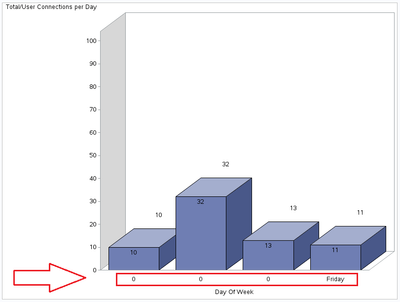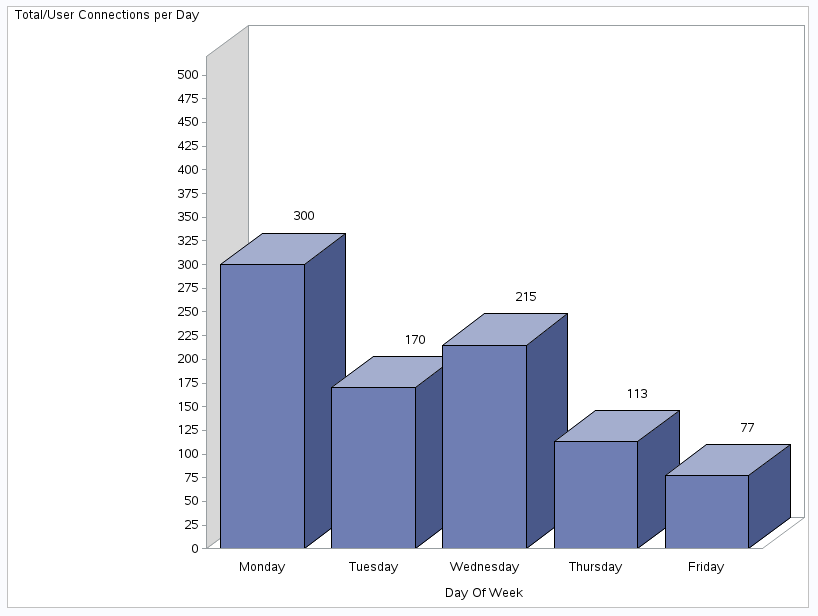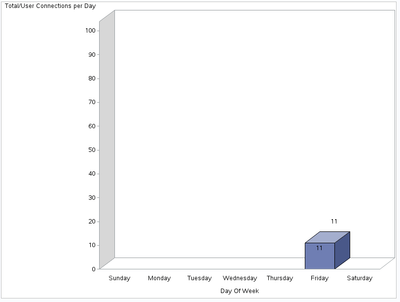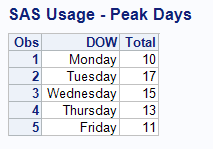## Proc gchart rounding the axis data

Hi,

Using the following data:

DOW        Total

------------------------
Monday     10
Tuesday    17
Wednesday  15
Thursday   13
Friday     11

The following sas code:

``````proc format;
value WDay 1='Sunday' 2='Monday' 3='Tuesday' 4='Wednesday' 5='Thursday' 6='Friday' 7='Saturday' other=0;
run;

ODS GRAPHICS on / RESET IMAGENAME="Fig1" IMAGEFMT=png ANTIALIASMAX=10000;
GOPTIONS RESET=ALL BORDER CBACK=WHITE HTITLE=12PT HTEXT=10PT;
OPTIONS CLEANUP;

Title1 'SAS Usage - Peak Days';
axis1 label=('Total/User Connections per Day') minor=none order=(0 to 100 by 10);
axis2 label=('Day Of Week');

legend1 label=(position=top) position=(right middle) across=1 shape=bar(.11in,.11in);

proc gchart data=TotalUsersPerDOW;
vbar3d DOW /
sumvar=Total
inside=sum
outside=sum
legend=legend1
width=20 space=16
raxis=axis1 maxis=axis2
;
run;quit;``````

produces the following:Why is the horizontal axis rounded? I expect to see data for Monday through Friday as my data set above clearly shows.

1 ACCEPTED SOLUTION

Accepted Solutions

## Re: Proc gchart rounding the axis data

S O L U T I O N

``````ODS GRAPHICS ON / RESET IMAGENAME="Fig1" IMAGEFMT=png ANTIALIASMAX=10000;
GOPTIONS RESET=ALL BORDER CBACK=WHITE HTITLE=12PT HTEXT=10PT;
OPTIONS CLEANUP;

proc format; value WDay 2='Monday' 3='Tuesday' 4='Wednesday' 5='Thursday' 6='Friday';run;
data junk;
input DOW Total;
datalines;
2 300
3 170
4 215
5 113
6 77
;

Title1 'SAS Usage - Peak Days';
axis1 minor=none label=('Total/User Connections per Day') order=(0 to 500 by 25);
axis2 minor=none label=('Day Of Week') order=(2 to 6 by 1);

proc gchart data=junk;
vbar3d DOW /
sumvar=Total
outside=sum
discrete
width=20 space=16
raxis=axis1 maxis=axis2
;
format dow Wday.;
run;quit;

ODS GRAPHICS OFF;
GOPTIONS RESET=ALL;``````An important property in GChart was also missing: 'discrete'

Special thanks to user 'BallardW' who contributed to this solution!

6 REPLIES 6

## Re: Proc gchart rounding the axis data

If you do not provide Values or Order on the Axis statement then the procedure will "pick" likely values for the axis.

Adjust your Axis2 statement with something like order=(1 to 7 by 1)

Or move to proc SGPLOT but the syntax will differ.

## Re: Proc gchart rounding the axis data

Hi Ballardw,

Thx for such a quick reply! I added 'order=(1 to 7 by 1)' but I now get:Am I missing a statment in 'gchart'?

## Re: Proc gchart rounding the axis data

It seems that there is an issue with the format, as if most of classes were recognized as part of the "other" values.

-> could you please test a simple proc print for example and apply the format to see if the "DOW" column is correctly displayed?

Best,

## Re: Proc gchart rounding the axis data

@ed_sas_member wrote:

It seems that there is an issue with the format, as if most of classes were recognized as part of the "other" values.

-> could you please test a simple proc print for example and apply the format to see if the "DOW" column is correctly displayed?

Best,

Nothing wrong with the format. The issue is understanding what Gchart does when there is not an explicit Order or Values specified for ticks. Likely the actual values used for the ticks were something close to 2.4, 3.6, 4.8 and 6.0 because the values in the data have the DOW of 2 (Monday) to 6(Friday);

```data junk;
input DOW        Total;
datalines;
2     10
3    17
4  15
5   13
6     11
;

proc gchart data=junk;
vbar3d DOW /
sumvar=Total
inside=sum
outside=sum
;
format dow f3.1;
run;quit;```

Without some instruction like ORDER= or Values= on the Axis Gchart will create groups based on the range of x-axis values seen. Only the "Friday" value in the data ends up with an integer in the range specified by the format. So everything else is "other".

## Re: Proc gchart rounding the axis data

Hi,

Here you go:## Re: Proc gchart rounding the axis data

S O L U T I O N

``````ODS GRAPHICS ON / RESET IMAGENAME="Fig1" IMAGEFMT=png ANTIALIASMAX=10000;
GOPTIONS RESET=ALL BORDER CBACK=WHITE HTITLE=12PT HTEXT=10PT;
OPTIONS CLEANUP;

proc format; value WDay 2='Monday' 3='Tuesday' 4='Wednesday' 5='Thursday' 6='Friday';run;
data junk;
input DOW Total;
datalines;
2 300
3 170
4 215
5 113
6 77
;

Title1 'SAS Usage - Peak Days';
axis1 minor=none label=('Total/User Connections per Day') order=(0 to 500 by 25);
axis2 minor=none label=('Day Of Week') order=(2 to 6 by 1);

proc gchart data=junk;
vbar3d DOW /
sumvar=Total
outside=sum
discrete
width=20 space=16
raxis=axis1 maxis=axis2
;
format dow Wday.;
run;quit;

ODS GRAPHICS OFF;
GOPTIONS RESET=ALL;``````An important property in GChart was also missing: 'discrete'

Special thanks to user 'BallardW' who contributed to this solution!

Discussion stats
• 6 replies
• 690 views
• 0 likes
• 3 in conversation